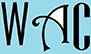## Algebra Question for Title 1 Schools: How Old Question?

6) Tim is 6 years older than Ron and Don is 5 years older than Tim, and their total age is 41.  How old is Ron?

t = 6 + r

d = 5 + t

t + r + d = 41

6 + r + r + 5 + 6 + r = 41

17 + 3r = 41

you have to make “r” subject of formula by moving  “17” to the other side, then “3”. When you go to the other side, addition becomes subtraction, multiplication becomes division, and vice versa.

3r = 41 – 17 (17 was addition at the other end)

3r = 24

r = 24/3 (3 was multiplication at the other end)

r = 8

Ron is 8 years old